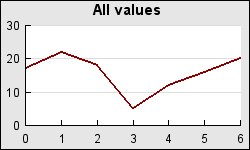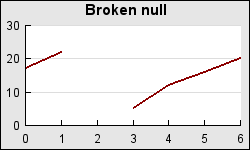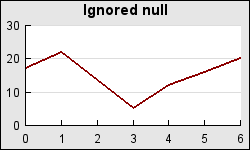## 13.6. Different types of NULL data handling

In some data there might be data points missing. For example if some experimental data is to be graphed there can be data points where the equipment was faulty and not valid values exist. The library offers to way to handle this.

1. Leave the data point empty and leave a whole in the graph, for example a break in a line plot. This happens iof the null value is specified as either one of the following values

• `'x'` - A single character 'x'

• `''` - An empty string

• `NULL` - The NULL value

2. Ignore the data point and connect the previous and next data point with the line in a line graph. This is accomplished by specifying the null value as

• `'-'` - A single hyphen character

In Example 13.1 an example with the different types of null values are shown. In these graphs the third data point is set to null with the two different null values.

Example 13.1. Illustration of different types of NULL values in graphs

 Figure 13.4. Original graph with all valuesFigure 13.5. Value at x=2 as ''Figure 13.6. Value at x=2 as '-'### Caution

If all values in a line plot are null values an error will be shown since this is not considered to be a valid plot.

Figure 13.7. Error when only null values are specified